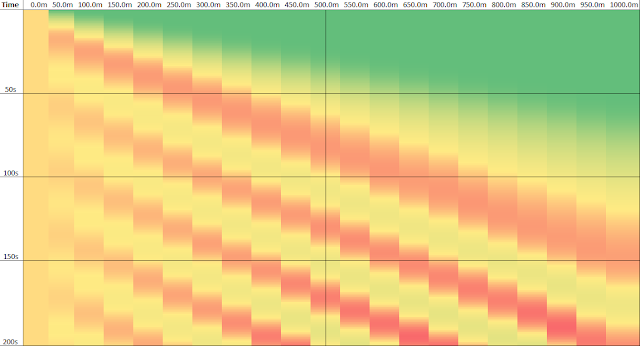## Saturday 2 February 2013

### Solving the Discretised Traffic Model Equations

This post follows on from when we discretised our traffic model equations. We now solve them in this post.

The solving process is quite simple since we avoided most references to the future values of variables. If we did, we'd have to use matrices to solve the discretised model equations. As it is, we can simply calculate each value separately.

The code is modeled around each cell, so here's the discretised cell equation: $$\rho(x_i,t_f) = \rho(x_i,t_c) - \frac{t_f-t_c}{x_{i+}-x_{i-}}\left(\rho(x_{i+},t_c) v(x_{i+},t_c) - \rho(x_{i-},t_c) v(x_{i-},t_c)\right).$$ where
$$\rho$$ = vehicular density (veh/m);
$$t$$ = time (s);
$$t_c$$ = the current time (s);
$$t_f$$ = the future time (s);
$$x$$ = distance (m) along road;
$$i$$ = the index of the current cell, ranging from 1 to $$n$$;
$$x_i$$ = the location of the 'centroid' of the current cell (m);
$$x_{i-}$$ = the left (upstream) boundary of the current cell
$$x_{i+}$$ =
$$v_f$$ = free flow speed (m/s);
$$\rho_j$$ = jam density (veh/m), the average vehicles per metre in stationary traffic; and
$$v$$ = vehicle speed (m/s), and is given by: $$v = v_f(1-\rho/\rho_j)$$.

The left boundary is given by: $$\rho(0,t) = \rho_j/4.$$

Oh, and in case it isn't clear, the flow of traffic is in the direction of increasing $$x$$ , which is to the right. That means that upstream is to the left, and downstream is to the right.

The above equations have been implemented in Common Lisp, with MathP (but I'll wait till the end to show the final code). It outputs the values scaled up by 1000 for convenience. To call it, we evaluate something like this:

(step-simulation :road-length 1000 :n 20 :dt 1.0 :time-run 200)

Great! So, let's look at the results. Here is a visualisation of the results for the above simulation:Initial traffic simulation results. Time increases from top to bottom, distance increases from left to right. Light traffic is green, medium traffic is yellow, and heavier traffic is red.

Not so good... there are fluctuations in the solution, causing negative vehicle densities. Thankfully, that can be fixed with an Upwind scheme. In our case, we just move the centroid more upstream within the cell. This removes the fluctuations, but probably increases error elsewhere in the simulation. The results with the Upwind scheme are:"Upwind" traffic simulation results. Time increases from top to bottom, distance increases from left to right. Light traffic is green, medium traffic is yellow, and heavier traffic is red.

Looking good. The traffic on the left fills the road over time, and there are no fluctuations.

The final code to implement this is:

(defparameter *free-flow-speed* (/ 60 3.6); = 60 km/h
"Speed (m/s) of freely flowing, low density traffic.")
(defparameter *jam-density* (/ 7.0); = 1 veh every 7 m

"Jam density (veh/m), the average vehicles per metre in stationary traffic.")
(defparameter *road-length* 1000.0; = 1 km

"Length (m) of road being simulated.")
(defparameter *cell-count* 10

"Number of cells road is split into for simulation.")

(defun step-simulation (&key
(vf *free-flow-speed*)
(pj *jam-density*)
(n *cell-count*)
(dt 1.0); Time step in seconds.
(time-run 10.0); Time to run simulation for.
(t0 0.0); Starting time for simulation.
(upwind 0.5); Upwinding parameter [0 = only upstream, 1 = only downstream]
p0; Initial road density for each cell.
pin); Input density function (veh/m). Should take one argument - the time.
#M{
; Spacing between cell centres.
pin = if pin pin fn(_) pj/4.0

;; Set the boundary density in the initial state.
unless(p0 p0 := makeArray(n :initialElement 0.0) p0 := #!pin(t0))
v(p) = vf*(1-p/pj); Velocity as function of density.
x(i) = (i-1)*dx; Cell centroid locations.
xp(i) = if i<n (i-0.5)*dx roadLength; Plus, or right (downstream) boundary locations.
xm(i) = if i>1 (i-1.5)*dx 0; Minus, or left (upstream) boundary locations.
stepsim(pold atime dt) =
makeVector(n fn(i) {
pm = if i>1 (1-upwind)*pold[i-2]+upwind*pold[i-1] pold
pp = if i<n (1-upwind)*pold[i-1]+upwind*pold[i] pold[n-1]
in = max(0 dt*pm*v(pm))
out= max(0 dt*pp*v(pp))
if i==1 #!pin(atime) pold[i-1] + (in - out)/(xp(i)-xm(i))})
#L
(loop for atime from t0 to (+ t0 time-run) by dt
for ps = p0 then (stepsim ps atime dt)
do (loop for p across ps
do (format t " ~4,1,3F" p)
finally (terpri)))})